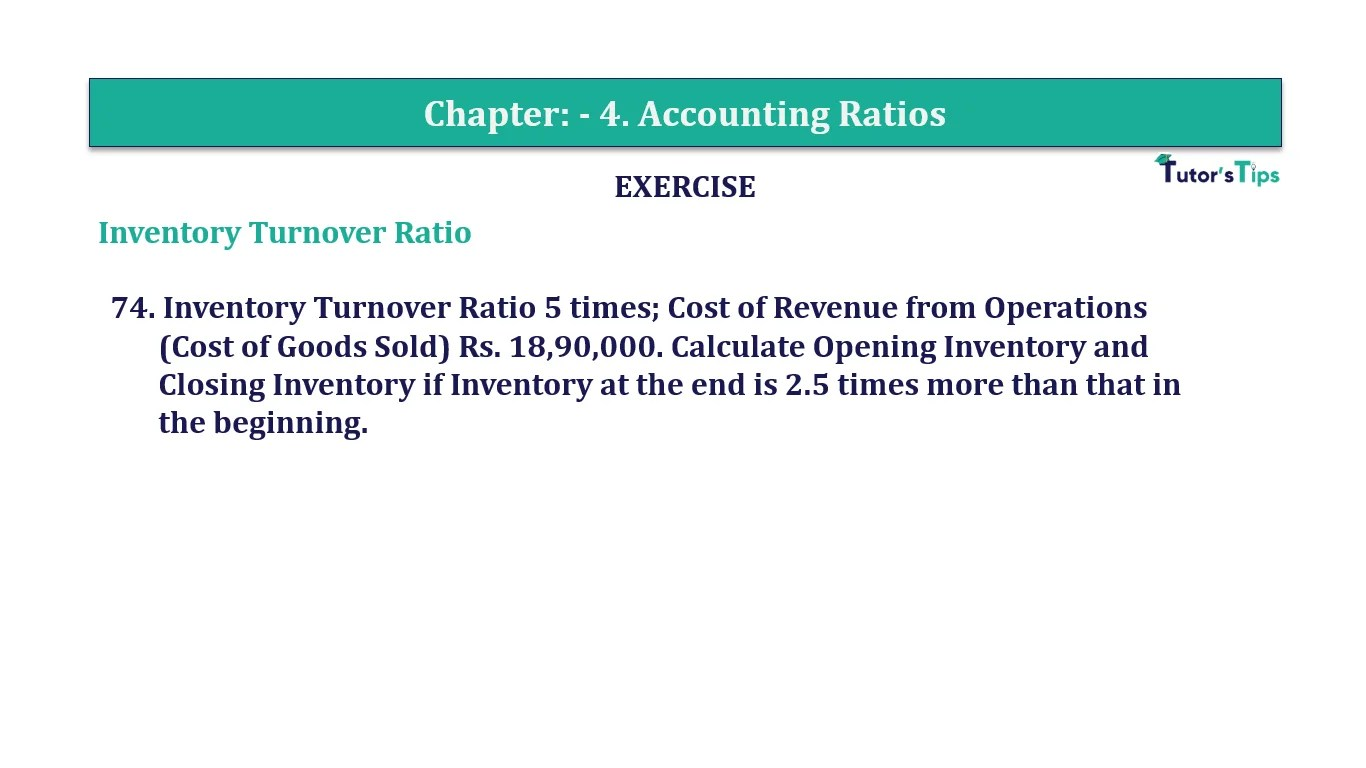# Question 74 Chapter 4 of +2-B – T.S. Grewal 12 ClassQuestion No. 74- Chapter No.4 - T.S. Grewal +2 Book Part B

Question 74 Chapter 4 of +2-B

Interest Coverage Ratio

74. Inventory Turnover Ratio 5 times; Cost of Revenue from Operations
(Cost of Goods Sold) Rs. 18,90,000. Calculate Opening Inventory and Closing Inventory if Inventory at the end is 2.5 times more than that in the beginning.

### The solution of Question 74 Chapter 4 of +2-B: –

 Inventory Turnover Ratio = Cost of goods sold Average Inventory
 5 = Rs. 18,90,000 Average Inventory Average Inventory = Rs. 18,90,000 / 5 Average Inventory = Rs. 3,78,000 Opening Inventory = X Closing Inventory = 2.5x + x

 Average Inventory = Opening Inventory + Closing Inventory 2
 Rs. 3,78,000 = X + (2.5x + X) 2

 4.5x = Rs. 7,56,000

 X = Rs. 7,56,000 4.5 = Rs. 1,68,000

 Opening Inventory = Rs. 1,68,000 Closing Inventory = 3.5 x Rs. 1,68,000 = Rs. 5,88,000

Balance Sheet: Meaning, Format & Examples

Comment if you have any question.

Also, Check out the solved question of previous Chapters: –